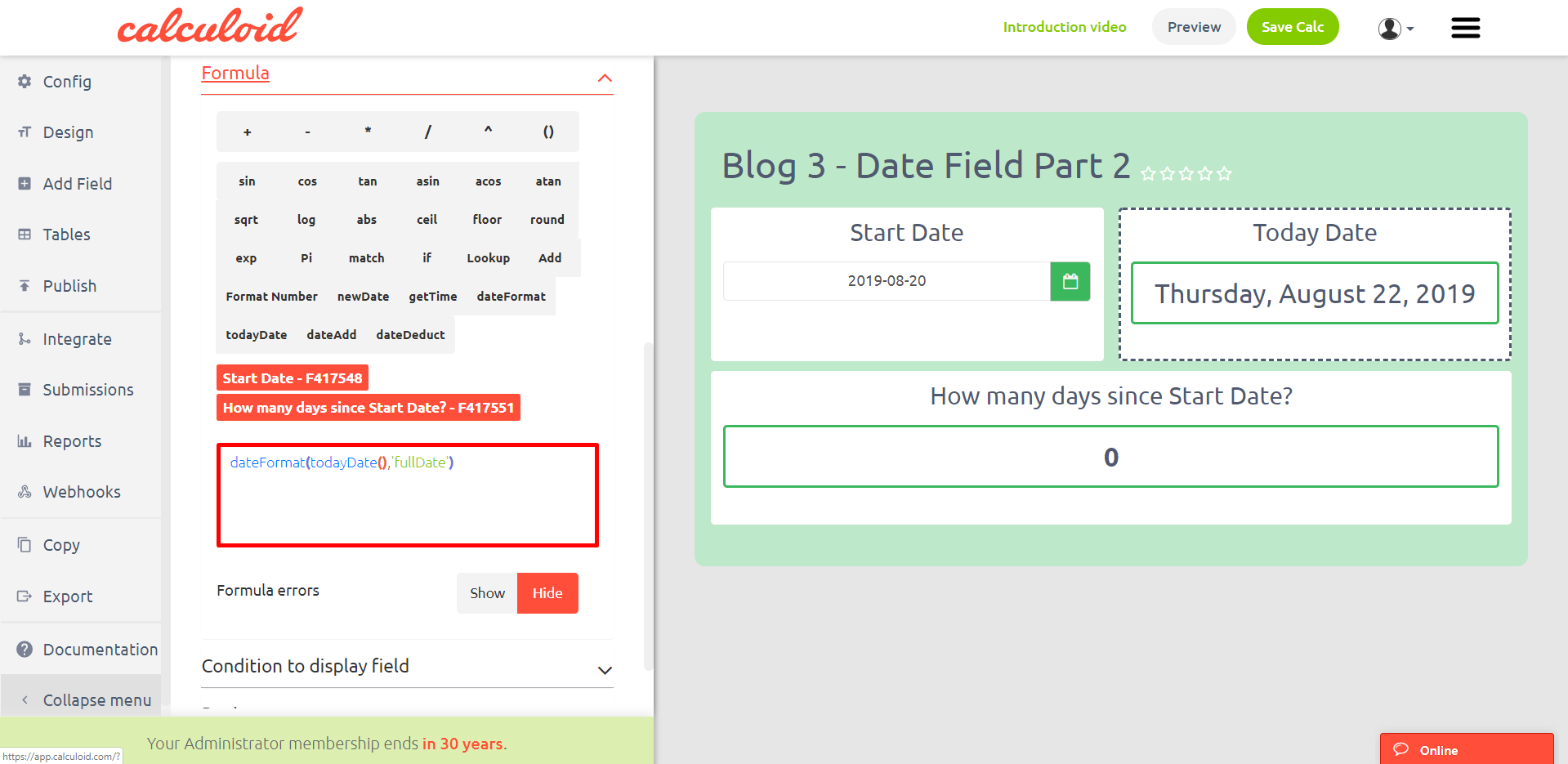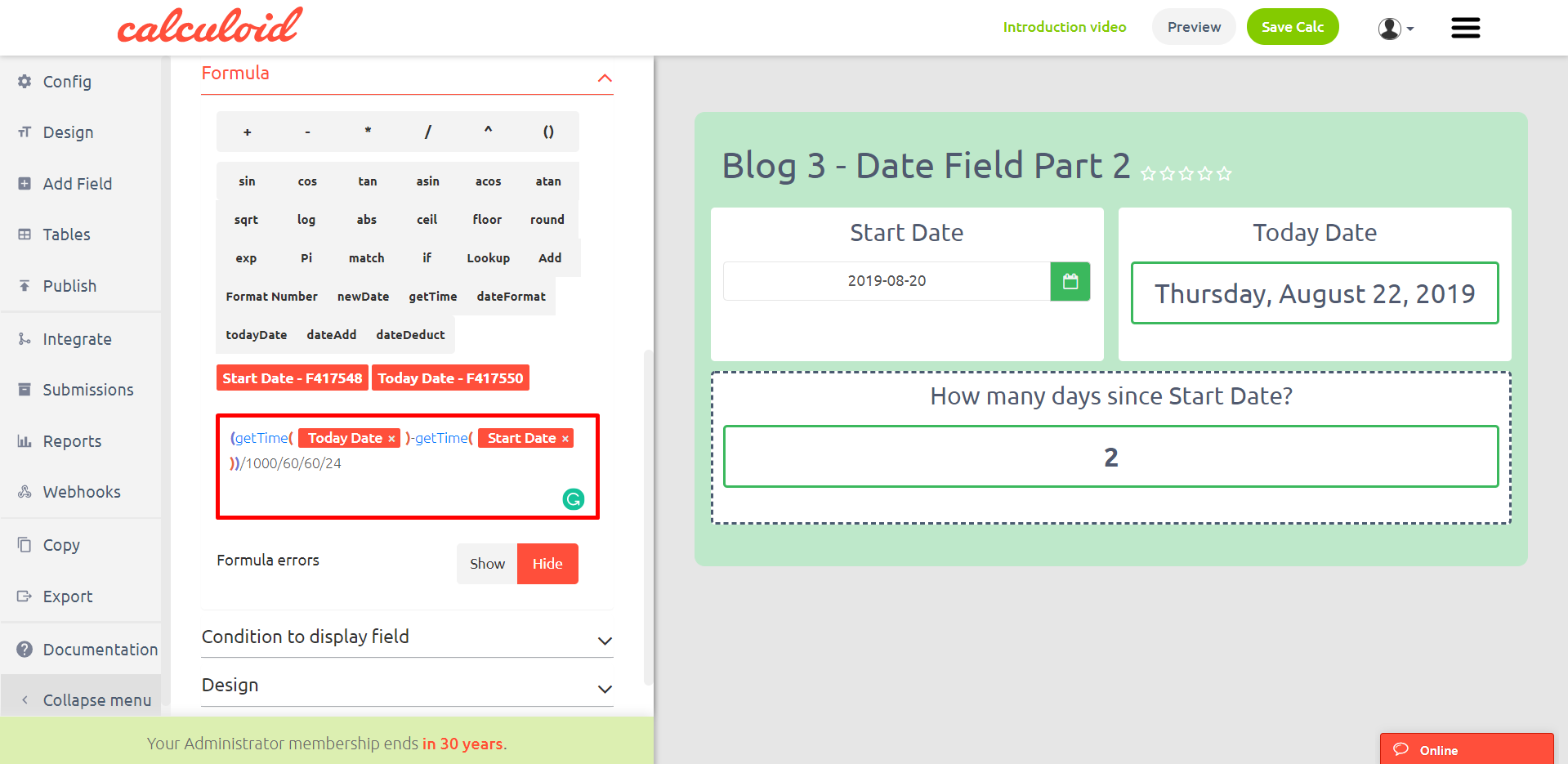Content

# Web calculators case studies #4 – Date field and functions Part 2

Date calculations are now available with Calculoid. We added Date field and 5 date functions to the formula field. Let's take a quick look at how can you use it in your calculators.

Explore case studies and get inspired on how to use Date field and functions to grow your business in Calculoid webinar recording.

Imagine creating a calculator that needs to calculate the difference between the starting date and today's date. All you need is to add a Date field to your calculator where the start date can be selected and two formula fields for today's date and further calculations.

One of the formula fields will be used for showing today's date using todayDate function. This is a better way than forcing your clients to select today's date manually. It is also nice to use formatDate function with todayDate to present the result in a custom format.

Description of todayDate function: todayDate().## Case study 1 – Two getTime functions to get the difference between two dates in days

To find the difference between two dates we need getTime function. This date function returns date in the absolute format presented in milliseconds.

Description of getTime function: getTime(date). Date can be taken from a date field or a formula field.

To calculate how many days are gone from the starting date till now we need to take today's date from the formula field and use getTime function. Then do the same with the date from the date field and take the second number from the first one.

Example: getTime (Today Date) - getTime (Start Date)The result is now in milliseconds. To present it in days we need to divide the number by 86,400,000.